# Long Division Calculator – with Steps to Solve

Enter the divisor and dividend below to calculate the quotient and remainder using long division. The results and steps to solve it are shown below.

Enter the divisor and dividend

## Result:

Full Answer: 18 R 3 18 3

### Solution

 1 8 4 7 5 - 4 3 5 - 3 2 3
Learn how we calculated this below

## How to do Long Division with Remainders

Learning long division is a crucial milestone in learning essential math skills and a rite of passage to completing elementary school. It strikes fear in elementary school students and parents alike.

A recent study found that the understanding of long division and fractions in elementary school is directly linked to the student’s ability to learn and understand algebra later in school.

Have no fear!

Learning long division can be easy, and in just a few easy steps, you can solve any long division problem. Follow along as we break it down, but first, we need to cover the anatomy of a long division problem.

### Parts of a Long Division Problem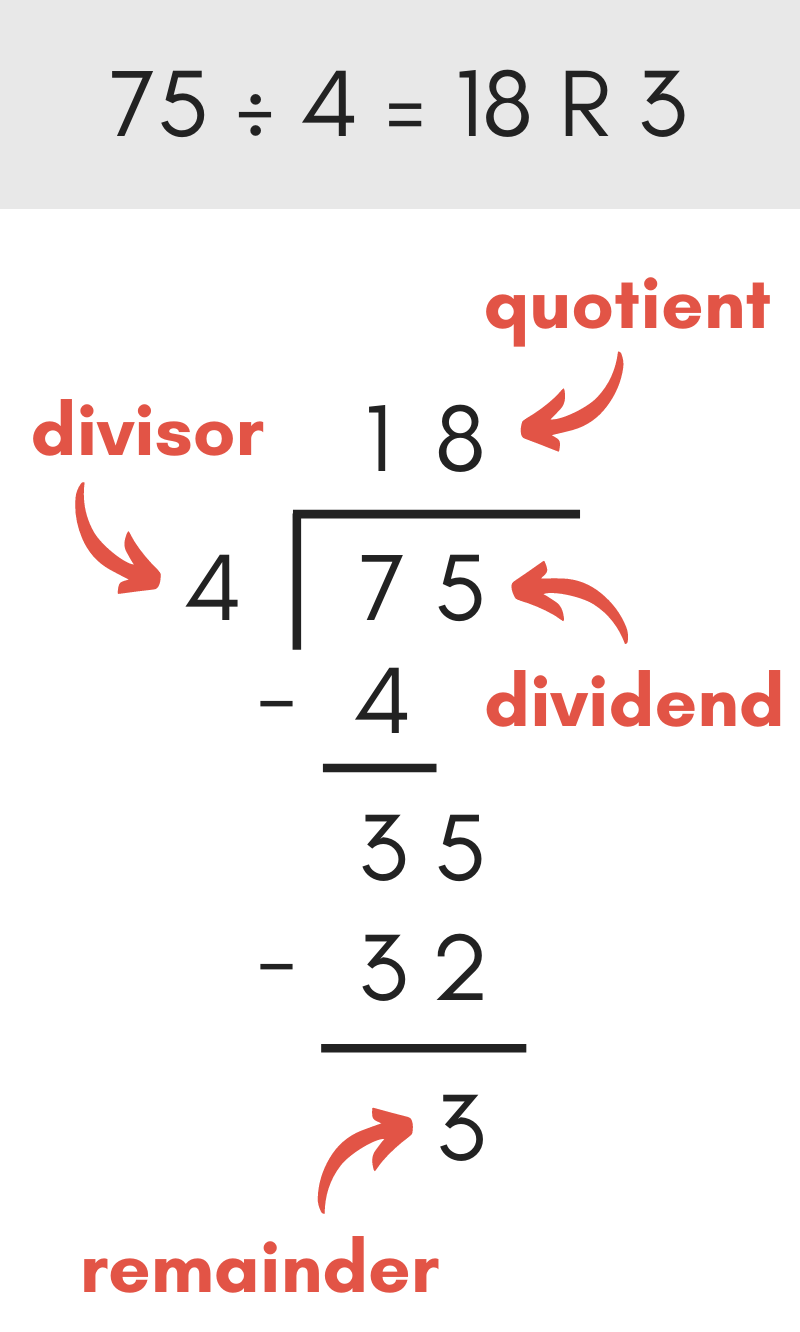There are a few parts to a long division problem, as shown in the image above.

The dividend is the number to the right and under the division line and is the number being divided.

The divisor is the number to the left of the division line and is the number being dividing by.

The quotient is the solution and is shown above the dividend over the division line. Often in long division, the quotient is the whole number part of the solution.

The remainder is the remaining part of the solution, or what’s leftover, that doesn’t fit evenly into the quotient.

### Steps to Calculate a Long Division Problem

There are a few main steps to solving a long division problem: divide, multiply, subtract, bringing the number down, and repeating the process.

#### Step One: Set up the Equation

The first step in solving a long division problem is to draw the equation that needs to be solved. If the problem is already in long division form, then skip along to step two.

If it’s not, this is how to draw the long division problem.

Start by drawing a vertical bar to separate the divisor and dividend and an overbar to separate the dividend and quotient.

Place the dividend to the right of the vertical bar under the overbar. Place the divisor to the left of the vertical bar.

For example, to divide 75 by 4, the long division problem should look like this: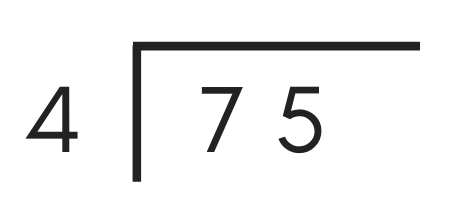#### Step Two: Divide

With the long division problem drawn, start by dividing the first digit in the dividend by the divisor.

You can also think about this as counting the number of times the divisor will fit into the digit in the dividend.

Drop the remainder or decimal portion of the result and write the whole number portion of the result in the quotient above the overline directly above the digit in the dividend.

For example, the divisor “4” goes into the first digit of the dividend “7” one time, so a “1” can be added to the quotient.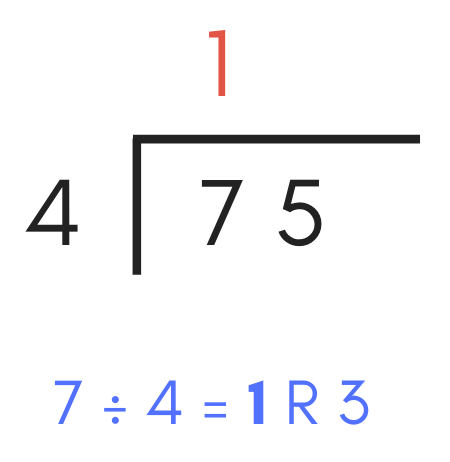#### Step Three: Multiply

The next step is to multiply the divisor by the digit just added to the quotient. Write the result below the digit in the dividend.

This step forms the next part of the equation for the next step.

Multiplying the divisor “4” by “1”, which we found in the previous step, equals “4”. So, add a “4” below the first digit in the dividend.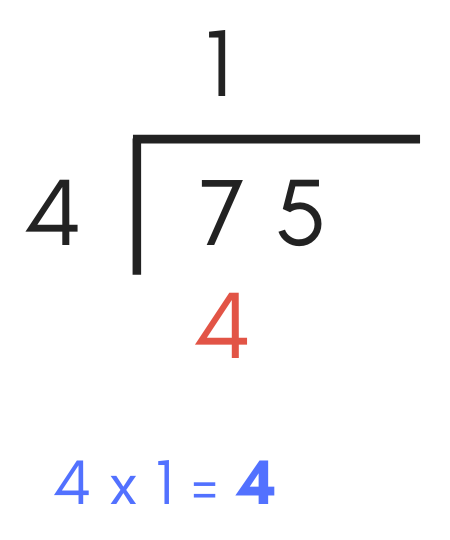#### Step Four: Subtract

Now, add a minus sign “-” before the number added in the previous step and draw a line below it to form a subtraction equation.

Continuing the example above, add a “-” before the “4” and a subtraction line below it.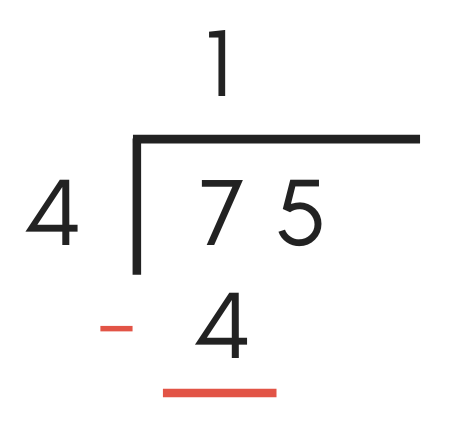Now that you have a subtraction problem created, it’s time to solve it.

To solve, subtract “7” minus “4”, which equals “3”, so write a “3” in the equation.If the long division problem has a dividend that is a single digit, then hooray, you’re done! The remaining number that is the result of the subtraction problem is the remainder, and the number above the dividend is the solved quotient.

If more digits are remaining in the dividend, then proceed to the next step.

#### Step Five: Pull Down the Next Number

At this point in the process, it’s time to operate on the next number in the dividend. To do this, pull down the next digit in the dividend and place it directly to the right of the result from the subtraction problem above.

The next digit in the dividend is “5”. So, pull “5” down and write it next to the “3” found in the previous step.#### Step Six: Repeat

At this point, you might be wondering where to go from here. Repeat steps two to five until all the digits in the dividend have been pulled down, divided, multiplied, and subtracted.

When dividing, use the result of the subtraction problem combined with the pulled down digit as the dividend and divide the divisor into it.

Continuing the examples above, divide the result of the subtraction problem and the pulled down digit by the divisor. Thus, the next step is to divide 35 by 4. The result is “8”, so add “8” to the quotient.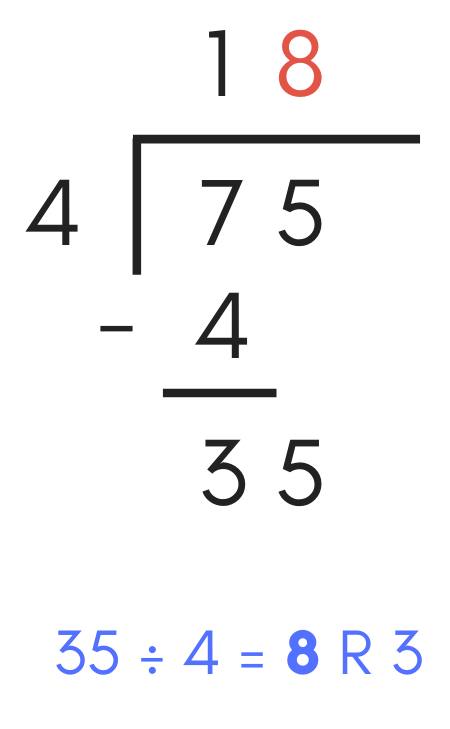Next, multiply the quotient digit “8” by the divisor “4”, which equals 32. Add “32” to the long division problem.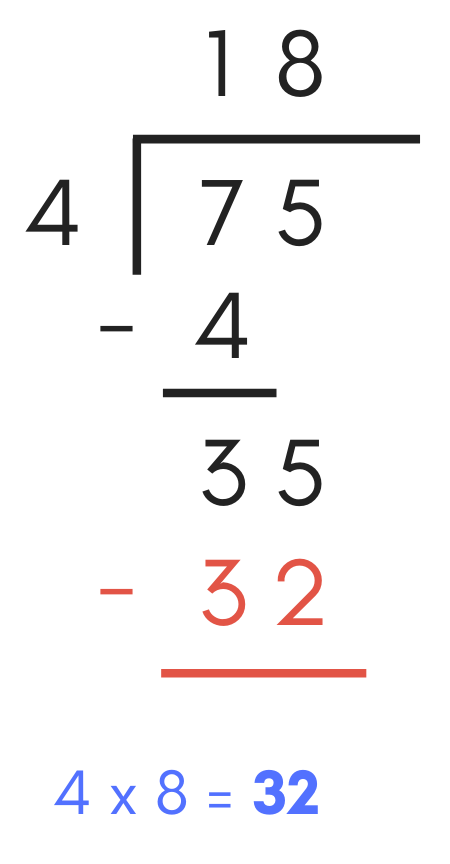Next, repeat the subtraction process, subtracting 32 from 35, which equals 3. Add a “3” below the subtraction line. Since there are no longer any remaining digits in the dividend, this is the remainder portion of the solution.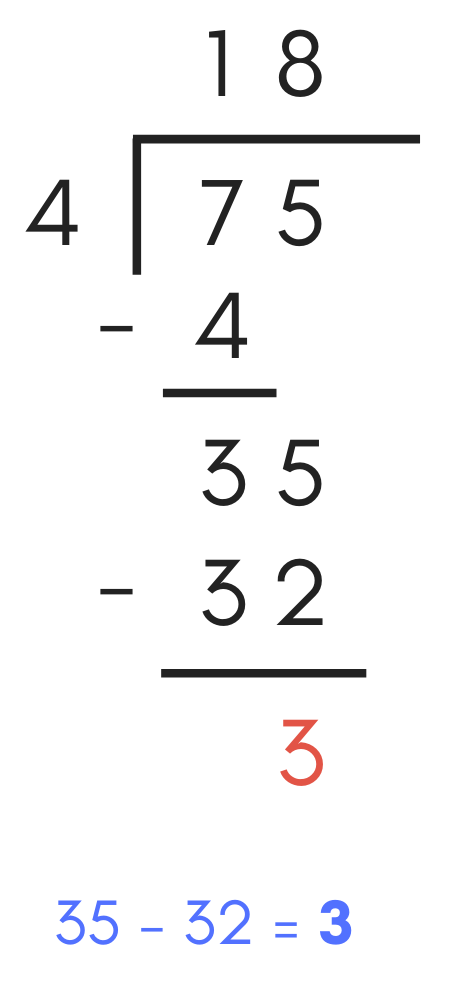As you practice these steps, use the calculator above to confirm your answer and validate your steps solving long division problems.

## How to get the Quotient and Remainder as a Decimal

If you’ve gotten this far then you should have a good idea of how to calculate a long division problem, but you might be stuck if you need to get the quotient as a decimal rather than a whole number with a remainder.

To calculate the quotient in decimal form, follow the steps above the get the whole number and remainder.

Next, divide the divisor by the remainder to get the remainder as a decimal. Finally, add the decimal to the quotient to get it in decimal form.

For example, 75 ÷ 4 is 18 with a remainder of 3.

Divide 3 by 4 to get the decimal 0.75.
3 ÷ 4 = 0.75

Then, add 0.75 to 18 to get the quotient as a decimal.
0.75 + 18 = 18.75

Thus, the decimal form of 75 ÷ 4 is 18.75.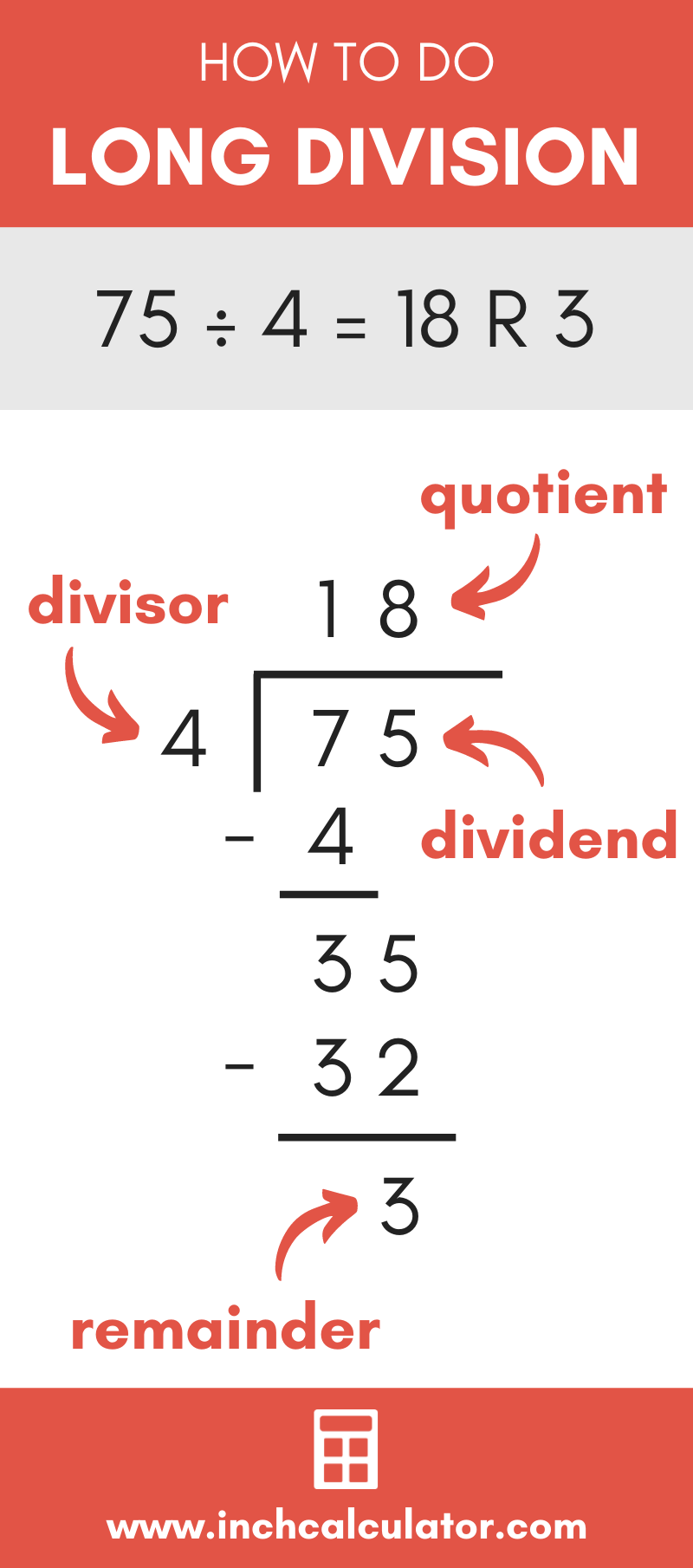## References

1. Carnegie Mellon University, Press Release: Carnegie Mellon-Led Research Team Finds Knowledge Of Fractions and Long Division Predicts Long-Term Math Success, https://www.cmu.edu/news/stories/archives/2012/june/june15_mathsuccess.html